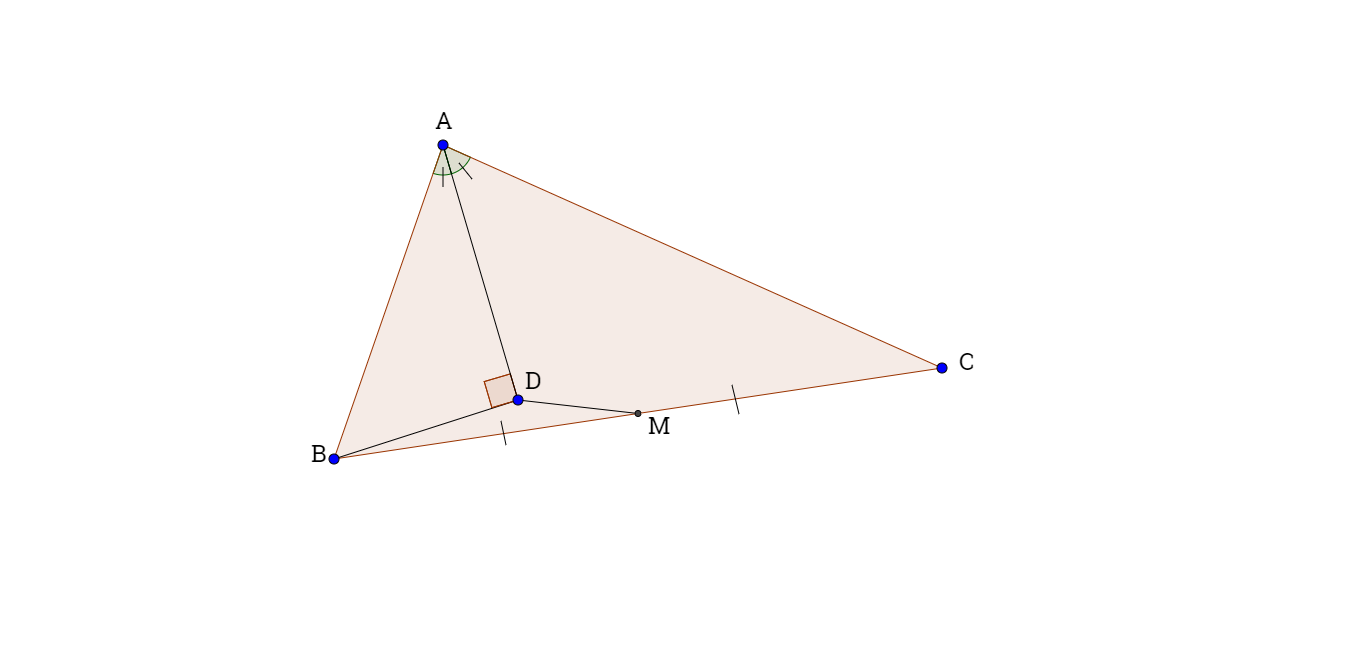# Right Triangle In Triangle

Geometry Level 5In the figure above, $\Delta ABC$ is a triangle. $\overline { AD }$ is the angle bisector of $\angle BAC$. Also $\angle BDA={ 90 }^{\circ}$ and $M$ is a point on $\overline {BC}$ so that $|\overline { BM } |=|\overline { MC } |$.

Given that $|\overline {AB}|=17$ and $|\overline {AC}|=61$, find $|\overline {DM} |$.

Note:

$|\overline {DM}|$ refers to the length of line segment DM.

$\overline {DM}$ refers to the line segment DM.

The diagram may not be drawn to scale.

×

Problem Loading...

Note Loading...

Set Loading...